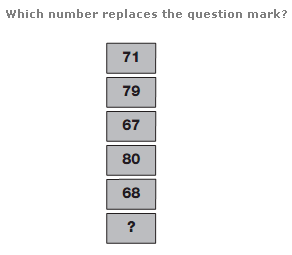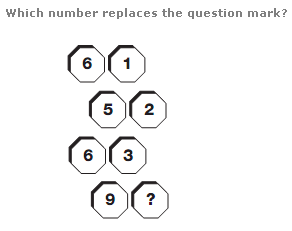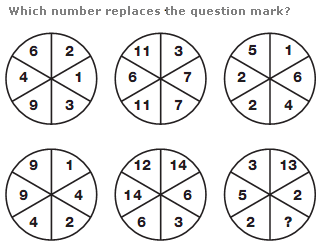# Puzzles - Number puzzles

### Exercise :: Number puzzlesAnswer : 82 Explanation : Start at the top and work down. Add the separate digits of the top number to the number itself to give the next one down, then subtract 12 to give the one after this. Continue with this alternating pattern.Answer : 4 Explanation : From top to bottom, reading each pair of numbers as a 2 digit value, the numbers follow the sequence of square numbers, from 16 to 49, each written back to front.Answer : 1 Explanation : In each row of the diagram, the values in the central circle equal the sums of the digits in corresponding segments of the left and right hand circles.